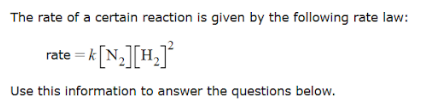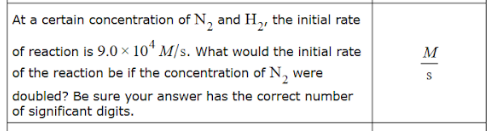# The rate of a certain reaction is given by the following rate law: rate = k[N2][H2]^2 Use this information to answer the questions below. At a certain concentration of N2 and H2, the initial rate of reaction is 9.0 x 0^4 M/s. What would the initial rate of the reaction be if the concentration of N2 were doubled? Be sure your answer has the correct number of significant digits.